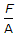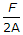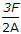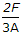# Civil Engineering - Theory of Structures

Exercise : Theory of Structures - Section 1
31.
A material is said to be perfectly elastic if
it regains its original shape on removal of the load
It regains its original shape partially on removal of the load
it does not regain its original shape at all
none of these.
Explanation:
No answer description is available. Let's discuss.

32.
For calculating the allowable stress of long columns. The empirical formula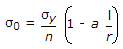, is known as
Straight line formula
Parabolic formula
Perry's formula
Rankine's formula.
Explanation:
No answer description is available. Let's discuss.

33.
Beams composed of more than one material, rigidly connected together so as to behave as one piece, are known as
Compound beams
Indeterminate beams
Determinate beams
Composite beams.
Explanation:
No answer description is available. Let's discuss.

34.
Pick up the correct statement from the following:
In a loaded beam, the moment at which the first yield occurs is called yield moment
In a loaded beam, the moment at which the entire section of the beam becomes fully plastic, is called plastic moment
In a fully plastic stage of the beam, the neutral axis divides the section in two sections of equal area
The ratio of plastic moment to the yield moment, is called shape factor
All the above.